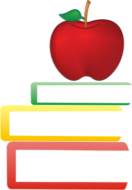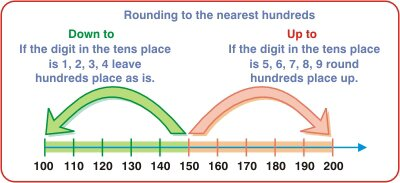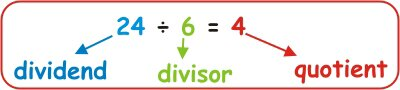# 3rd Grade Math Learning - Math Worksheets and Activities - MyHomeSchoolMath

myhomeschoolmath.comTitle
Go to content## 3rd Grade Math Learning - Math Worksheets and Activities

Free printable Math Worksheets - 3rd grade
This is a collection of math worksheets for grade 3, organized by topics such as counting, comparing, rounding, place value, addition, subtraction, multiplication, division, fractions, measuring, and geometry.
The worksheets are, except in pdf form, randomly generated each time you click on the links below. You can also get a new, different one just by refreshing the page in your browser.
Numbers, Numbers sequences, Comparing numbers, Rounding, Place valueSubtraction
MultiplicationDivisionMixed operations - Order of operations
Dynamically created worksheets
Equations with variables
Dynamically created worksheets
Fractions
Dynamically created worksheets
Decimals
Dynamically created worksheets
Learning Metric system
Dynamically created worksheets
Learning Roman numerals
Dynamically created worksheets
Geometry
Back to content